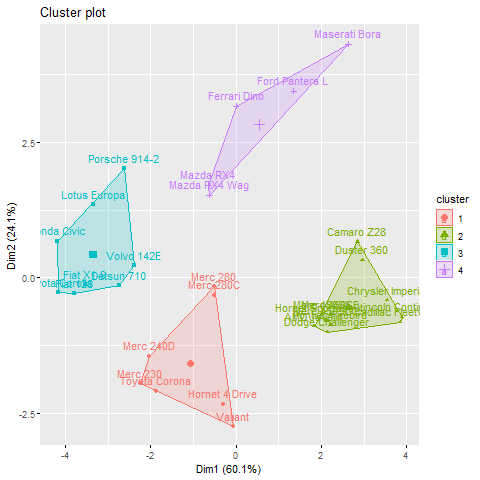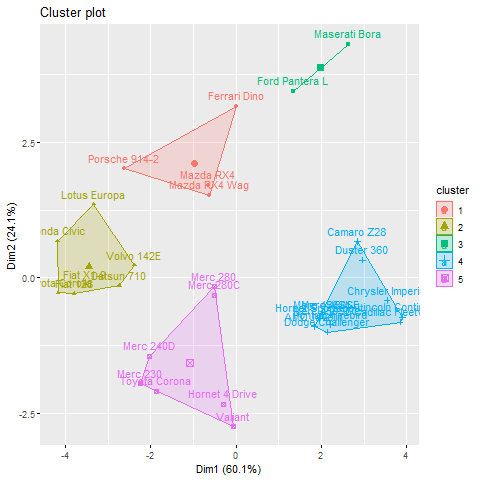GFG App
Open AppBrowser
Continue

Clustering in R Programming Language is an unsupervised learning technique in which the data set is partitioned into several groups called as clusters based on their similarity. Several clusters of data are produced after the segmentation of data. All the objects in a cluster share common characteristics. During data mining and analysis, clustering is used to find similar datasets.

## Applications of Clustering in R Programming Language

• Marketing: In R programming, clustering is helpful for the marketing field. It helps in finding the market pattern and thus, helping in finding the likely buyers. Getting the interests of customers using clustering and showing the same product of their interest can increase the chance of buying the product.
• Medical Science: In the medical field, there is a new invention of medicines and treatments on a daily basis. Sometimes, new species are also found by researchers and scientists. Their category can be easily found by using the clustering algorithm based on their similarities.
• Games: A clustering algorithm can also be used to show the games to the user based on his interests.
• Internet: An user browses a lot of websites based on his interest. Browsing history can be aggregated to perform clustering on it and based on clustering results, the profile of the user is generated.

## Methods of Clustering

There are 2 types of clustering in R programming:

• Hard clustering: In this type of clustering, the data point either belongs to the cluster totally or not and the data point is assigned to one cluster only. The algorithm used for hard clustering is k-means clustering.
• Soft clustering: In soft clustering, the probability or likelihood of a data point is assigned in the clusters rather than putting each data point in a cluster. Each data point exists in all the clusters with some probability. The algorithm used for soft clustering is the fuzzy clustering method or soft k-means.

## K-Means Clustering in R Programming language

K-Means is an iterative hard clustering technique that uses an unsupervised learning algorithm. In this, total numbers of clusters are pre-defined by the user and based on the similarity of each data point, the data points are clustered. This algorithm also finds out the centroid of the cluster.

Algorithm:

• Specify number of clusters (K): Let us take an example of k =2 and 5 data points.
• Randomly assign each data point to a cluster: In the below example, the red and green color shows 2 clusters with their respective random data points assigned to them.
• Calculate cluster centroids: The cross mark represents the centroid of the corresponding cluster.
• Re-allocate each data point to their nearest cluster centroid: Green data point is assigned to the red cluster as it is near to the centroid of red cluster.
• Re-figure cluster centroid

Syntax:  kmeans(x, centers, nstart)

where,

• x represents numeric matrix or data frame object
• centers represents the K value or distinct cluster centers
• nstart represents number of random sets to be chosen

Example:

## R

 # Library required for fviz_cluster function install.packages("factoextra") library(factoextra)   # Loading dataset df <- mtcars   # Omitting any NA values df <- na.omit(df)   # Scaling dataset df <- scale(df)   # output to be present as PNG file  png(file = "KMeansExample.png")   km <- kmeans(df, centers = 4, nstart = 25)   # Visualize the clusters fviz_cluster(km, data = df)   # saving the file  dev.off()   # output to be present as PNG file  png(file = "KMeansExample2.png")   km <- kmeans(df, centers = 5, nstart = 25)   # Visualize the clusters fviz_cluster(km, data = df)   # saving the file  dev.off()

Output:

When k = 4When k = 5## Clustering by Similarity Aggregation

Clustering by similarity aggregation is also known as relational clustering or Condorcet method which compares each data point with all other data points in pairs. For a pair of values A and B, these values are assigned to both the vectors m(A, B) and d(A, B). A and B are the same in m(A, B) but different in d(A, B).where, S is the cluster

With the first condition, the cluster is constructed and with the next condition, the global Condorcet criterion is calculated. It follows in an iterative manner until specified iterations are not completed or the global Condorcet criterion produces no improvement.

My Personal Notes arrow_drop_up